# 7 Solving The Initial Value Problem Using Cartan Calculus

Y. Fourès

#### DOI

10.34663/9783945561294-12

#### Citation

Fourès, Y. (2011). Solving The Initial Value Problem Using Cartan Calculus. In: The Role of Gravitation in Physics: Report from the 1957 Chapel Hill Conference. Berlin: Max-Planck-Gesellschaft zur Förderung der Wissenschaften.

I write for the space-time manifold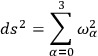7.1

where I take as the basis in my four-dimensional manifold one vector orthogonal to the initial hypersurface, so that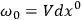7.2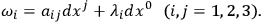7.3

Then the differential relations for the field can be written in the form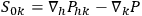7.4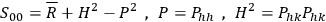7.5

where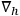is the covariant derivative, and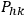is what Lichnerowicz in the orthogonal calls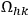. (For further notation see Section 5.4 of the talk by Lichnerowicz earlier in this session.) If the coordinates were orthogonal we would have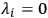, and hence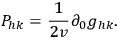7.6

The coefficients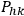are the coefficients of the second fundamental quadratic form of the hypersurface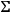.

We are dealing with the purely gravitational case. To solve the differential equations I choose on the hypersurface three particular vectors, the eigenvector of the tensor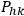, that is to say we will have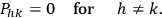7.7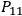,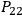,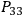are unknowns. The metric of the hypersurface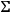is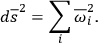7.8

If I set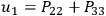, et cycl., and if I use Lichnerowicz’s idea to take the metric on the hypersurface conformal to a given metric, denoted by an asterisk: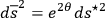7.9

then I obtain the following system of equations: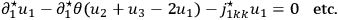7.1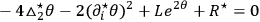7.11

where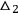is the Beltrami operator of second order, and where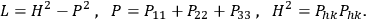7.12

“It is possible to solve this system of equations which are linear with respect to the highest derivatives. For instance, I can solve them by giving the values of the unknown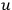in my three-dimensional space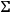on a two-dimensional variety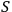, and I can solve then the Cauchy problem for the system which contains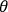. A general iteration method can be used to solve the set of equations by writing them in the form of integral equations using Green’s functions. If I give the value ofon the boundary, and the values of the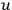on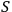, then I find the general solution of these equations. This is a rigorous mathematical treatment of the problem of initial values.”

WHEELER pointed out the analogy with the electromagnetic case where the equation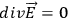yields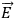in all space if it is known over a two-dimensional surface. However, one would still like to have a potential which gives the field automatically without having to do any integration.

MME. FOURES, answering a question of MISNER, pointed out that the statement that the metric is conformal to a Euclidean one is an assertion, not an assumption, and that her method gives general solutions for an open space.

DE WITT asked if the Cauchy problem is now understood sufficiently to be put on an electronic computer for actual calculation. “Do we now know enough about constraints and initial conditions to do this at least for certain symmetrical cases?”

MISNER replied that you could take some of the particular solutions which he has given, and which start out as instantaneously static fields, or the interesting example of two particles which start to rotate about each other, and you could perform actual calculations. “You start out with a Euclidean metric and would use the method of Mme. Fourès’ proof.1 On the surface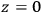you put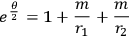7.13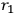and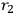being distances measured from two points in space. Then you assign initial conditions for the eigenvalues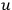. You want to specifyin such a way that the velocities of the two particles are initially like that: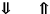Then you try to use the same method which was used for the general proofs to find particular solutions not just on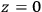but through all space. These can be interpreted as two particles which are non-singular, or they can be thought of as the kind of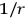type singularity of which one ordinarily thinks in gravitational theory. These partial differential equations, although very difficult, can then in principle be put on a computer.” MISNER thinks that one can now give initial conditions so that one would expect to get gravitational radiation, and computers could be used for this.

DE WITT pointed out some difficulties encountered in high speed computational techniques. “Singularities are of course difficult to handle. Secondly, any non-linear hydrodynamic calculations are always done in so-called Lagrangian coordinates, so that the mesh points move with the material instead of being fixed in space. Similar problems would arise in applying computers to gravitational radiation since you don’t want the radiation to move quickly out of the range of your computer.”

BERGMANN remarked on the general discussion of the afternoon that six years ago it was discovered that one can find a closed form for the Hamiltonian of the Einstein equations, and it was thought then that all troubles with the Cauchy problem for gravitational theory were over. “This is not so, for the simple reason that there are eight constraints for the twenty canonical variables. Of the remaining twelve variables eight have nothing to do with the problem, they correspond to an arbitrariness in the choice of coordinates, so that one is left with four canonical variables. Can you introduce new variables such that these variables could be set up arbitrarily? This is essentially a search for potentials in the general sense in which that term has been used here.” BERGMANN is personally convinced that the search for locally defined potentials is probably hopeless, because they probably do not exist. “This is only a belief, of course.”

ANDERSON commented on the problem of two-surface boundary conditions. He suggested that since it is possible to put the gravitational Lagrangian into a form in which the time derivatives of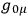do not appear, just as the time derivative of the scalar potential does not appear in the electromagnetic Lagrangian, it may be possible to break up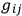in a fashion analogous to the separation of the vector potential in the electromagnetic case into transverse and longitudinal parts. Only one of these parts appears in those equations of motion which are free from second time derivatives. One could then initially and finally specify the part which does not appear in these equations, and obtain a complete solution. There may be one catch to this. There is reason to believe that one of the field equations, which do not depend on second time derivatives, is really the Hamiltonian of the theory. If that is the case one would not be allowed to specify independent initial and final values for the quantities just discussed.

Discussion of these points, which are closely related with the problem of quantization, was postponed until later.

WHEELER returned to the central problem of the afternoon, the initial value problem. He asked whether, leaving technical details aside, there is a point of view which would permit us to find our way around some of these subtleties.

“I would like to raise in this connection the question of the use of the variational principle itself. The variational principle in general relativity has a character rather different from that in electromagnetic theory. In the latter we have the square of the field variables, whereas in the gravitational case we have the curvature, i.e., the second derivatives of the potentials entering. This has always puzzled us: Why is the variational formulation of the gravitational field so different? I would like to emphasize that the convenience which one so often has sought in transforming by partial integration the second derivatives into first derivatives has concealed what the equations are trying to tell us. I would like to illustrate this by a trivial example. Take the free particle of ordinary mechanics. The variational principle is: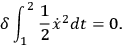7.14

How do we tell what to fix at the endpoints of the time interval? We do this by performing an integration by parts: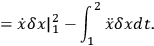7.15

From this we draw two separate conclusions:

1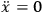.

2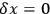at the two endpoints of the motion, i.e., we must specify the coordinate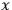at the times 1 and 2. This is a time-symmetric specification. One is so accustomed to this pattern of thinking that when general relativity presents us with a different pattern we are not prepared for that. Therefore, let me introduce a problem which does have the pattern characteristic of general relativity, and let us see how we would face up to it. Consider this: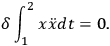7.16

Traditionally one abhors these second derivatives in the problem, so one quickly transforms it into the previous problem. I propose to deal rather directly with the new problem like this: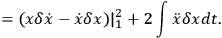7.17

The conclusions would now be:

1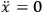.

2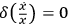, i.e., we are to specify the value of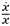at the two endpoints of the motion.

“In other words, we are led here to a different kind of specification of the problem. I merely want to point out that the variational problem itself has the property if we don’t monkey with it, to tell us what it is that we should deal with.”

“In the case of electromagnetism we can also let the variational principle tell us what the quantity is that we are concerned with. Note the equation:2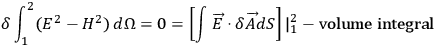7.18

The volume integral gives us the usual field equations. The boundary term tells us that we should specify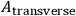at the limits. If you specify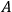itself, rather than its transverse part, you would be taking too seriously the demand that the boundary term must vanish. Clearly you don’t have to be that hard on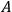. Analogous considerations can and have been applied to the general relativity by Misner and Fletcher.”

MISNER outlined his present, tentative results on this question. He noted that one has not yet been able to specify the gravitational variables which are analogous to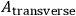in the sense mentioned by Wheeler. “With arguments based on the normalization in Feynman integration one can single out the invariant variational principle: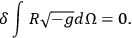7.19

From this one gets the surface term of form: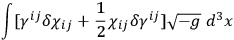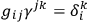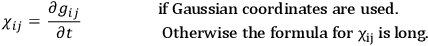The suggestion is that if you look at the surface term carefully you will find what the correct variables to use are, and what you can specify on the initial and final surface.”

MISNER summarized the discussion of this session: “First we assume that you have a computing machine better than anything we have now, and many programmers and a lot of money, and you want to look at a nice pretty solution of the Einstein equations. The computer wants to know from you what are the values of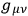and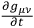at some initial surface, say at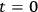. Now, if you don’t watch out when you specify these initial conditions, then either the programmer will shoot himself or the machine will blow up. In order to avoid this calamity you must make sure that the initial conditions which you prescribe are in accord with certain differential equations in their dependence on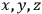at the initial time. These are what are called the “constraints.” They are the equations analogous to but much more complicated than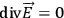. They are the equations to which we have been finding particular solutions; and on the other hand, Mme. Fourès has shown the existence of more general kinds of solutions. Mme. Fourès has told us that to get these initial conditions you must specify something else on a two-dimensional surface and hand over that problem, the problem of the initial values, to a smaller computer first, before you start on what Lichnerowicz called the evolutionary problem. The small computer would prepare the initial conditions for the big one. Then the theory, while not guaranteeing solutions for the whole future, says that it will be some finite time before anything blows up.”

LICHNEROWICZ emphasized that one seeks to answer the question whether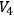is compact and whether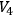is orientable.

## Footnotes

J. Rat. Mech. Anal. 2, 951 (1956).

We are using the notation of Landau and Lifshitz, Classical Theory of Fields.